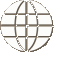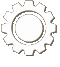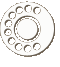COURSE Material
Course picture not available.
Foundations of Mathematics 20

Foundations of Mathematics 20 is divided into seven units. It meets the required outcomes outlined by Saskatchewan Learning, and is a prerequisite for Foundations of Mathematics 30. Students will require a TI-83 or TI-84 calculator in order to complete this course. This workbook series was developed for use by schools using an individualized, mastery-based learning system, and therefore comes with score keys, tests, and test keys. No additional text is necessary.

Unit 1: Inductive and Deductive Reasoning

At the end of unit one, the student should be able to:

• understand the difference between inductive and deductive reasoning
• form and analyze conjectures
• prove or disprove conjectures to solve problems
• solve problems using inductive and deductive reasoning
• analyze and strategize puzzles involving spatial reasoning

Unit 2: Properties of Angles and Triangles

At the end of unit two, the student should be able to:

• determine the measure of unknown angles using properties of angles formed by parallel lines and transversals
• determine the measure of unknown angles using angle properties of triangles
• determine the measure of unknown angles using angle properties of polygons
• derive proofs based on theorems and postulates about parallel lines and triangles
• solve problems that involve angles, parallel lines, transversals, and triangles

Unit 3: Proportional Reasoning

At the end of unit three, the student should be able to:

• solve problems that involve rates
• solve problems that involve scale factors
• determine the relationship between scale factors, surface areas, and volumes of similar objects
• use the relationship between scale factor, surface area, and volume in similar objects to solve problems
• solve real world problems using the properties of similar triangles

Unit 4: Systems of Linear Inequalities

At the end of unit four, the student should be able to:

• graph linear inequalities
• solve problems using linear inequalities in two variables
• solve problems using systems of inequalities in two variables
• graph the feasible region of a system of linear inequalities
• optimize a given objective quantity
• solve word problems by optimizing the objective quantity

Unit 5: Quadratic Functions

At the end of unit five, the student should be able to demonstrate an understanding of the characteristics of quadratic functions of the form y = a(x - p)2 + q, including:

• vertex
• intercepts
• domain and range
• axis of symmetry

Unit 6: Trigonometry

At the end of unit six, the student should be able to:

• completely solve a right triangle
• understand angle of elevation and angle of depression
• use the law of sines to solve triangles (determine side lengths and angle measures)
• use the law of cosines to solve triangles (determine side lengths and angle measures)
• solve problems by applying the law of sines and the law of cosines
• determine how many triangles are possible in the ambiguous case of an SSA triangle

Unit 7: Statistical Reasoning

At the end of unit seven, the student should be able to:

• analyze a numerical data set by creating a grouped frequency distribution table, a histogram, and a frequency polygon
• use a graphing calculator to analyze data as above
• calculate the mean, median, and the mode of a numerical data set
• calculate the range, mean deviation, and standard deviation of a numerical data set
• understand what it means for data to be normally distributed
• use a normal curve to estimate the number of data items lying within a specified range
• use a graphing calculator to draw normal curves
• convert actual data items to z-scores
• find the area under the standard normal curve
• find the probability of events whose outcomes are normally distributed
• use the area under the standard normal curve to estimate an actual data value
COURSE MaterialsSAICS has developed Christian curriculum (where none other was available) to match Saskatchewan Learning objectives for high school courses.

View Our Course Listing
ORDERING CoursesFind out more information about ordering courses that have been developed by SAICS including score keys and associated textbooks.

Ordering Information